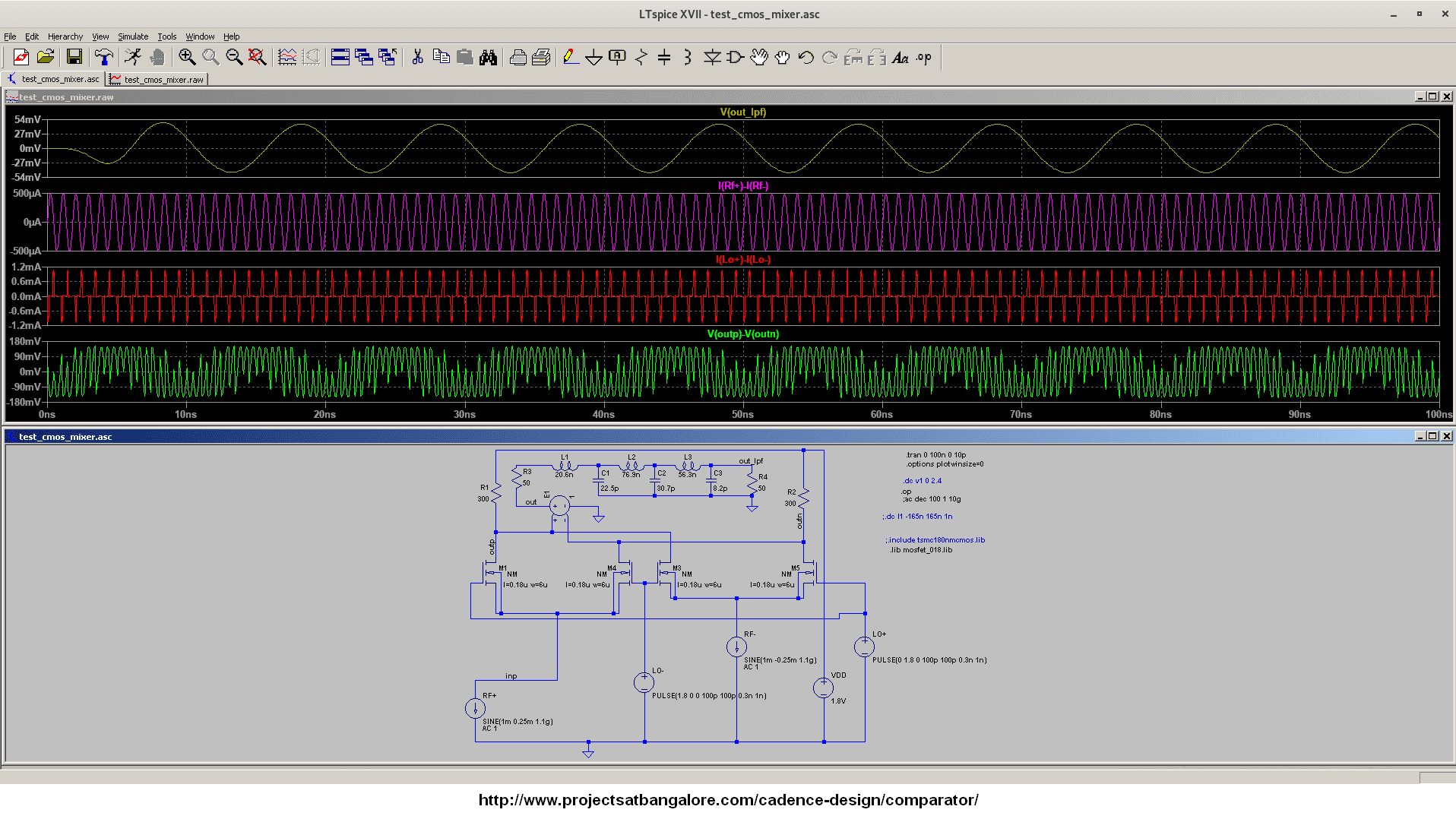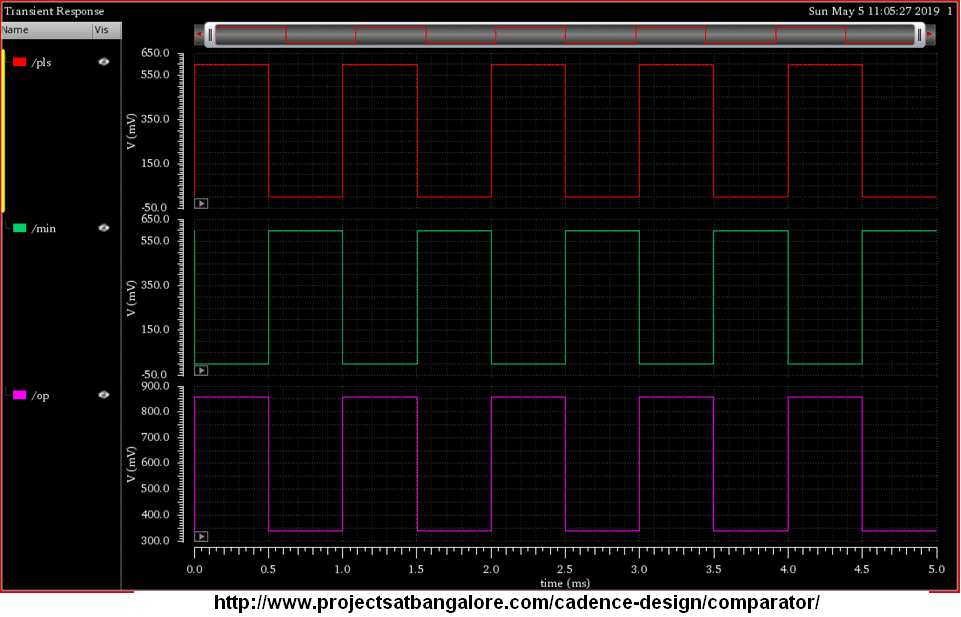Call:9591912372The Op-amp comparator compares one analogue voltage level with another analogue voltage level, or some preset reference voltage, VREF and produces an output signal based on this voltage comparison. In other words, the op-amp voltage comparator compares the magnitudes of two voltage inputs and determines which is the largest of the two.

With reference to the op-amp comparator circuit above, lets first assume that VIN is less than the DC voltage level at VREF, ( VIN < VREF ). As the non-inverting (positive) input of the comparator is less than the inverting (negative) input, the output will be LOW and at the negative supply voltage, -Vcc resulting in a negative saturation of the output.

If we now increase the input voltage, VIN so that its value is greater than the reference voltage VREF on the inverting input, the output voltage rapidly switches HIGH towards the positive supply voltage, +Vcc resulting in a positive saturation of the output. If we reduce again the input voltage VIN, so that it is slightly less than the reference voltage, the op-amp’s output switches back to its negative saturation voltage acting as a threshold detector.

### Positive and Negative Voltage Comparators

A basic op-amp comparator circuit can be used to detect either a positive or a negative going input voltage depending upon which input of the operational amplifier we connect the fixed reference voltage source and the input voltage too. In the examples above we have used the inverting input to set the reference voltage with the input voltage connected to the non-inverting input.

But equally we could connect the inputs of the comparator the other way around inverting the output signal to that shown above. Then an op-amp comparator can be configured to operate in what is called an inverting or a non-inverting configuration.

• #### Positive Voltage Comparator

The basic configuration for the positive voltage comparator, also known as a non-inverting comparator circuit detects when the input signal, VIN is ABOVE or more positive than the reference voltage, VREF producing an output at VOUT which is HIGH as shown.

• #### Negative Voltage Comparator

The basic configuration for the negative voltage comparator, also known as an inverting comparator circuit detects when the input signal, VIN is BELOW or more negative than the reference voltage, VREF producing an output at VOUT which is HIGH as shown.